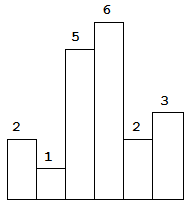# 84. Largest Rectangle in Histogram (Hard)

https://leetcode.com/problems/largest-rectangle-in-histogram/

Given n non-negative integers representing the histogram's bar height where the width of each bar is 1, find the area of largest rectangle in the histogram.Above is a histogram where width of each bar is 1, given height = `[2,1,5,6,2,3]`.The largest rectangle is shown in the shaded area, which has area = `10` unit.

Example:

```Input: [2,1,5,6,2,3]
Output: 10
```

## Solutions

``````class Solution {

// This problem can be solved by a few lines of code. However, it is not a problem
// that can be thought through the problem-solving process readily. The idea for this
// problem is ingenious and pretty much like the problem 239. Sliding Window Maximum (Hard).

// This problem is different from 11. Container With Most Water (Medium). Problem 11
// requires to sort out the lowest boundary of the container so that we can work out the max
// volume of it.

// To sort out the maximum area, the brute-force solution is to go through all the possible sub
// arrays. Then find out the lowest histogram for each range.

// We apply a stack to keep elements that larger than stack top, which means we can always
// get the lowest histogram of a given range. For instance, stack holds elements [2,3,6] annd
// current position is 7, which means when we perform backtracking:

// 1. for any histogram bars after position 6 (7 is not guarantee, only sure for processed
// elements), the lowest is heights;

// 2. for histogram bars after position 3, the lowest becomes heights; You may think range
// [4:6] and [5:6] are neglected, however, we do it intentionally. Because they are already
// calculated, that is the reason why 4 and 5 are absent from the stack.

// 3. a little different for position 2, it represent the lowest bar from current to 0. Because
// position 0 and 1 should be higher then 2, otherwise they will be put into the stack.

public int largestRectangleArea(int[] heights) {
if (heights == null || heights.length == 0) {
return 0;
}

int len = heights.length;

//Once the index are determined, the shadow area depends on the lowest value
// within these two index.

Stack<Integer> stack = new Stack<>();

int max = 0;
for (int i = 0; i < len; i++) {

// if the current histogram is lower than previous one
while (!stack.isEmpty()) {
// Do not push in heights[i] if heights[i] < stack top because it breach
// the ascending order. Deal with elements on the stack top until it is able to meet
// forementioned requirements.
if (heights[stack.peek()] < heights[i]) {
break;
}

// Is there any chance that certain pair of continuous elements in stack is discrete
// in the histogram? The answer is yes.

// Pop out the element that of higher histogram bar than i
int higherHist = stack.pop();

int width = stack.isEmpty() ? i : (i - higherHist);

// From i (exclusive) to each element in stack, the lowest height is itself.
int height = heights[higherHist];

// Calculate the area of histograms from i to stack top.
int area = width * height;

max = Math.max(max, area);
}

// Elements push into stack are sorted naturally in ascending order.
stack.push(i);
}

while (!stack.isEmpty()) {
int area = heights[stack.pop()] * (stack.isEmpty() ? len : len - stack.peek() - 1);
max = Math.max(max, area);
}

return max;
}
}
``````